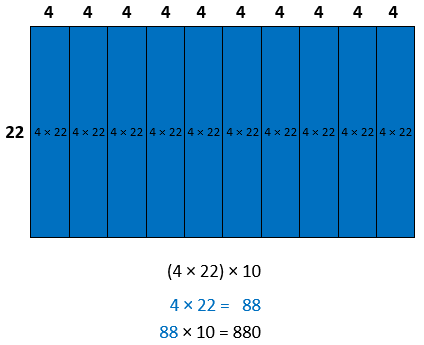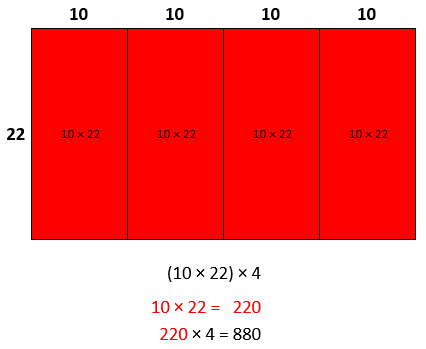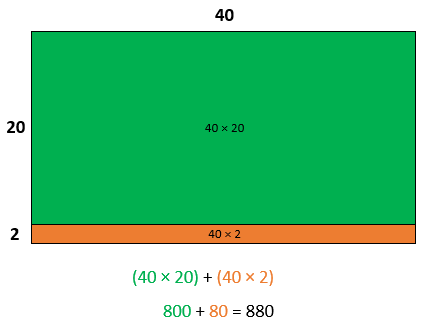# Multi-Digit Multiplication

## Objective

Multiply two-digit multiples of 10 by two-digit numbers.

## Common Core Standards

### Core Standards

?

• 4.NBT.B.5 — Multiply a whole number of up to four digits by a one-digit whole number, and multiply two two-digit numbers, using strategies based on place value and the properties of operations. Illustrate and explain the calculation by using equations, rectangular arrays, and/or area models.

?

• 3.NBT.A.3

• 4.NBT.A.1

• 4.NBT.B.4

• 3.OA.B.5

• 3.OA.C.7

## Criteria for Success

?

1.  Multiply multiples of 10 by two-digit numbers by decomposing the multiply of 10 and using the associative property (e.g., 80 x 34 = 8 x 10 x 34 = 340 x 8 or 272 x 10).
2. Multiply multiples of 10 by two-digit numbers by decomposing the two-digit number and using the distributive property (e.g., 80 x 34 = (80 x 30) + (80 x 4) = 2,400 + 320 = 2,720).

## Tips for Teachers

?

#### Remote Learning Guidance

If you need to adapt or shorten this lesson for remote learning, we suggest prioritizing Anchor Task 1 and Anchor Task 2 (benefit from discussion). Find more guidance on adapting our math curriculum for remote learning here.

#### Fishtank Plus

• Problem Set
• Student Handout Editor
• Vocabulary Package

?

### Problem 1

Two different students solved the problem 40 x 22 below. Study their work and determine whether the strategy they used works.### Problem 2

Yet another student solved $40 \times 22$ with a different strategy below. Study their work and determine whether the strategy they used works.### Problem 3

Estimate each product. Then use any method to solve.

a. 60 x 31

b. 18 x 70

c. 90 x 56

d. 35 x 80

## Problem Set & Homework

#### Discussion of Problem Set

• How is #1a of the Problem Set less complex than the others?
• How could you have used #3b to solve #4b?
• How could you have used #3c to solve #4c?
• Can you explain why #4c and #4d have the same product?
• When we record partial products, do we have to start with the one with the smallest place value? Will we get a different result if we start with the tens?
• When we multiply by a multiple of 10, why is there always a 0 in the ones place?

?

### Problem 1

Which of these expressions can be used to find the product 20 x 41? Select the two correct answers.

A.      41 x 10 + 2

B.      41 x 2 x 10

C.      (40 + 20) x (1 + 20)

D.       20 x 40 + 1

E.        41 x 2 x 10

F.        (20 x 40) + (20 x 1)

### Problem 2

Solve. Show or explain your work.

32 x 60

### Mastery Response

?